# 大家好!帮忙看看这个EA的代码为什么不会开仓?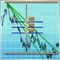488

若有侵权,本人愿意删除止贴.

//+------------------------------------------------------------------+

//|                                                  Combo_Right.mq4 |
//|                               Copyright ?2008, Yury V. Reshetov |
//+------------------------------------------------------------------+

//---- input parameters
extern double       tp1 = 50;
extern double       sl1 = 50;
extern int          p1 = 10;
extern int          x12 = 100;
extern int          x22 = 100;
extern int          x32 = 100;
extern int          x42 = 100;
extern double       tp2 = 50;
extern double       sl2 = 50;
extern int          p2 = 20;
extern int          x13 = 100;
extern int          x23 = 100;
extern int          x33 = 100;
extern int          x43 = 100;
extern double       tp3 = 50;
extern double       sl3 = 50;
extern int          p3 = 20;
extern int          x14 = 100;
extern int          x24 = 100;
extern int          x34 = 100;
extern int          x44 = 100;
extern int          p4 = 20;
extern int          pass = 1;
extern double       lots = 0.01;
extern int          mn = 888;
static int          prevtime = 0;
static double       sl = 10;
static double       tp = 10;

//+------------------------------------------------------------------+
//| expert start function                                            |
//+------------------------------------------------------------------+
int start()
{
if (Time == prevtime) return(0);
prevtime = Time;

again();
return(0);
}
//----
int total = OrdersTotal();
for (int i = 0; i < total; i++) {
if (OrderSymbol() == Symbol() && OrderMagicNumber() == mn) {
return(0);
}
}

sl = sl1;
tp = tp1;

int ticket = -1;

RefreshRates();

if (Supervisor() > 0) {
ticket = OrderSend(Symbol(), OP_BUY, lots, Ask, 1, Bid - sl * Point, Bid + tp * Point, WindowExpertName(), mn, 0, Blue);
if (ticket < 0) {
again();
}
} else {
ticket = OrderSend(Symbol(), OP_SELL, lots, Bid, 1, Ask + sl * Point, Ask - tp * Point, WindowExpertName(), mn, 0, Red);
if (ticket < 0) {
again();
}
}
//-- Exit --
return(0);
}
//+--------------------------- getLots ----------------------------------+

double Supervisor() {
if (pass == 4) {
if (perceptron3() > 0) {
if (perceptron2() > 0) {
sl = sl3;
tp = tp3;
return(1);
}
} else {
if (perceptron1() < 0) {
sl = sl2;
tp = tp2;
return(-1);
}
}
}

if (pass == 3) {
if (perceptron2() > 0) {
sl = sl3;
tp = tp3;
return(1);
} else {
}
}

if (pass == 2) {
if (perceptron1() < 0) {
sl = sl2;
tp = tp2;
return(-1);
} else {
}

}
}

double perceptron1()   {
double       w1 = x12 - 100;
double       w2 = x22 - 100;
double       w3 = x32 - 100;
double       w4 = x42 - 100;
double a1 = Close - Open[p2];
double a2 = Open[p2] - Open[p2 * 2];
double a3 = Open[p2 * 2] - Open[p2 * 3];
double a4 = Open[p2 * 3] - Open[p2 * 4];
return(w1 * a1 + w2 * a2 + w3 * a3 + w4 * a4);
}

double perceptron2()   {
double       w1 = x13 - 100;
double       w2 = x23 - 100;
double       w3 = x33 - 100;
double       w4 = x43 - 100;
double a1 = Close - Open[p3];
double a2 = Open[p3] - Open[p3 * 2];
double a3 = Open[p3 * 2] - Open[p3 * 3];
double a4 = Open[p3 * 3] - Open[p3 * 4];
return(w1 * a1 + w2 * a2 + w3 * a3 + w4 * a4);
}

double perceptron3()   {
double       w1 = x14 - 100;
double       w2 = x24 - 100;
double       w3 = x34 - 100;
double       w4 = x44 - 100;
double a1 = Close - Open[p4];
double a2 = Open[p4] - Open[p4 * 2];
double a3 = Open[p4 * 2] - Open[p4 * 3];
double a4 = Open[p4 * 3] - Open[p4 * 4];
return(w1 * a1 + w2 * a2 + w3 * a3 + w4 * a4);
}

return(iCCI(Symbol(), 0, p1, PRICE_OPEN, 0));
}

void again() {
prevtime = Time;
Sleep(30000);
}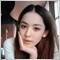799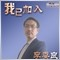2525

insane007: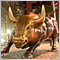4940

10年无一单亏损，还不是小手数死扛，这个EA逻辑也比较简单，不会那么神的。。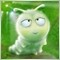14753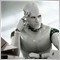296